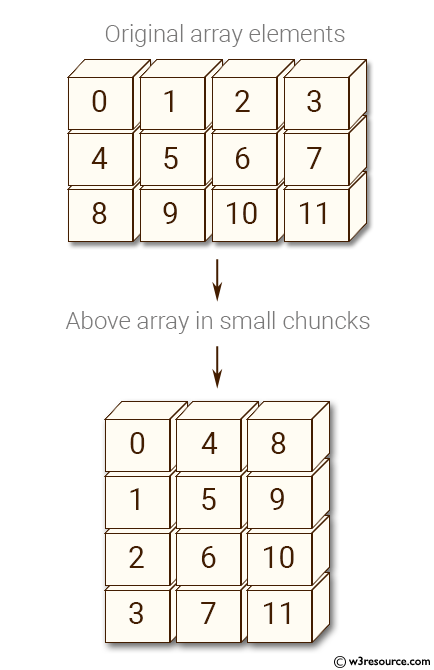﻿ NumPy: Create an array of (3, 4) shape and convert the array elements in smaller chunks - w3resource# NumPy: Create an array of (3, 4) shape and convert the array elements in smaller chunks

## NumPy: Array Object Exercise-77 with Solution

Write a NumPy program to create an array of (3, 4) shape and convert the array elements in smaller chunks.

Pictorial Presentation:Sample Solution:-

Python Code:

``````import numpy as np
x= np.arange(12).reshape(3, 4)
print("Original array elements:")
print(x)
print("Above array in small chuncks:")
for a in np.nditer(x, flags=['external_loop'], order='F'):
print(a)
```
```

Sample Output:

```Original array elements:
[[ 0  1  2  3]
[ 4  5  6  7]
[ 8  9 10 11]]
Above array in small chuncks:
[0 4 8]
[1 5 9]
[ 2  6 10]
[ 3  7 11]
```

Python Code Editor:

Have another way to solve this solution? Contribute your code (and comments) through Disqus.

What is the difficulty level of this exercise?

Test your Python skills with w3resource's quiz

﻿

## Python: Tips of the Day

Set comprehension:

```>>> m = {x ** 2 for x in range(5)}
>>> m
{0, 1, 4, 9, 16}
```